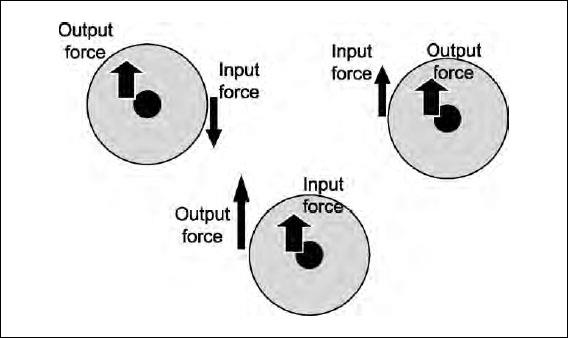# How is a wheel and axle similar to a lever?

What is a wheel and axle? It consists of a disk (the wheel) attached to a thin rod (the axle) so that the two rotate together. Typically the input force is applied to the outer edge of the wheel, and the output force is exerted on something, like a rope, attached to the outer edge of the axle. If the two forces are exerted in the same direction, then it is like a third-class lever. If the two are in the opposite direction (for example, a person pushing down on one side of the wheel while a rope is pulled up on the other side), then it is like a first-class lever. One may also have the input force exerted on the axle, in which case it is like a second-class lever.

Both the lever and the wheel and axle are really torque, not force, multipliers. Recall that if the force is at right angles to the line from the axis of rotation to the point where the force is applied, then the torque is given by Fr. Therefore, if the radius of the axel is a and the radius of the wheel is w, then if the input force is applied to the wheel, the output force is given by Foutput = Finput (w/a).Close

This is a web preview of the "The Handy Physics Answer Book" app. Many features only work on your mobile device. If you like what you see, we hope you will consider buying. Get the App